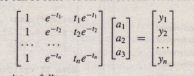Response of a Biomedical Instrument

Biomedical instrumentation is an important engineering field. These devices are used to measure many quantities. such as ‘body  temperature. blood oxygen level. heart rate. and so forth. Engineers developing such devices often need to obtain a response curve that describes how fast the instrument can make measurements. The theory of instrumentation shows that often the response can be described by one of the following equations. where
v is the voltage output. and t is time. In both. models. the voltage reaches a steady-state constant value as I -+ 00. and T is the time required for the voltage to equal 95 percent of the steady-state value.The following data gives the output voltage of a certain device as a function of time. Obtain a function that describes this data.• Solution
Plotting the data we estimate that it takes approximately 3 seconds for the voltage to become constant. Thus we estimate that T = 3. The first-order model writtenwhich can be solved for the coefficient vector a using left division. The following MATLAB script solves the problem.
t [0,0.3,0.8,1.1,1.6,2.3,3];
y [0,0.6,1.28,1.5,1.7,1.75,1.8];
X [ones(size(t)) ;exp(-t)]’;
a X\y’ The answer is QI = 2.0258 and Q2 = -1.9307.
A similar procedure can be followed for the second-order model.Continue the previous script as follows.
X = [ones(size(t));exp(-t);t.*exp(-t)]’;
a = X\y’
– I The answer is QI = 1.7496, Q2 = -1.7682, and Q3 = 0.8885. The two models are plotted with the data in Figure 5.6-8. Clearly the second-order model gives the better fit.